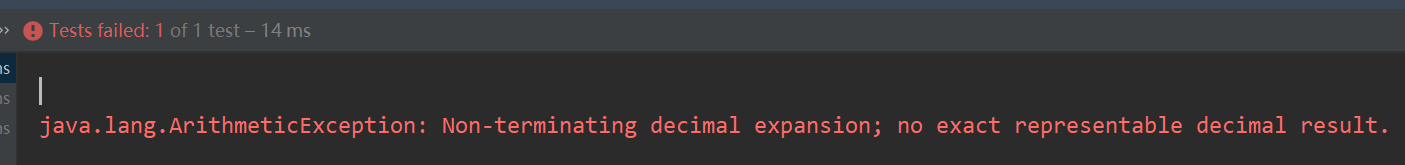• 累计撰写 213 篇文章
• 累计创建 36 个标签
• 累计收到 2 条评论

### 目 录CONTENT# BigDecimal知识回顾2022-02-11 / 0 评论 / 0 点赞 / 759 阅读 / 1,591 字 / 正在检测是否收录...

## 介绍

Bigdecimal是java.math包下的一个类，不可变的、任意精度的有符号十进制数，BigDecimal类使用户能完全控制舍入行为，如果需要精确计算的结果，则必须使用BigDecimal

BigDecimal 的等值比较应使用 compareTo()方法，而不是 equals()方法

    @Test
public void bigDecimal1(){
BigDecimal h = new BigDecimal("0.00");
BigDecimal k = BigDecimal.ZERO;
System.out.println(h.compareTo(k)==0);
System.out.println(h.equals(k));

}

true
false


## BigDicimal的加减乘除

@Test
public void bigDecimal(){
BigDecimal a = new BigDecimal("2.12");
BigDecimal b = new BigDecimal("0.2");
//加法
// 减法
BigDecimal d = a.subtract(b);
// 乘法
BigDecimal e = a.multiply(b);
double ff = e.doubleValue(); // 转换成double类型的值
// 除法,
BigDecimal f = a.divide(b);
}



   @Test
public void bigDecimal1(){
BigDecimal h = new BigDecimal(1.0);
BigDecimal k = new BigDecimal(0.9);
BigDecimal test = h.subtract(k);
System.out.println(test);
}## java开发手册规约

【强制】禁止使用构造方法 BigDecimal(double)的方式把 double 值转化为 BigDecimal 对象

Double 的 toString，而 Double 的 toString 按 double 的实际能表达的精度对尾数进行了截断。
BigDecimal recommend1 = new BigDecimal("0.1");
BigDecimal recommend2 = BigDecimal.valueOf(0.1);

## 用double进行小数的加减乘除会有什么问题

  @Test
public void doubleTest(){
double a = 1.0;
double b = 0.9;
double c = a - b;
System.out.println(c);
}


0